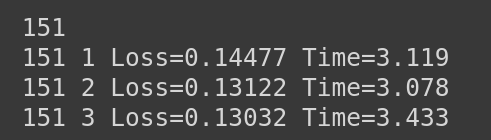Hi all, I am trying to implement a recent ICCV paper in PyTorch. I have to train an enormous sized dataset(in 10s of GB) for very large number of epochs(4000). So I am trying to store the model and optimizer state after certain epochs to save the state and load it afterwards.

``````torch.save({
'epoch': epoch,
'model_state_dict': net.state_dict(),
'optimizer_state_dict': optimizer.state_dict(),
'loss': loss
}, path)
``````

``````net = model(*args)
net = net.to(device)
optimizer = optim.Adam(net.parameters(), lr = 8e-5)
if train_epoch != None:
train_epoch = checkpoint['epoch']
loss = checkpoint['loss']
``````

I am observing that the loss from the loaded model if is 0.0756, when I start training with the loaded model, the loss after 1st iteration in the next epoch jumps to 0.23105.
It would take some number of epochs at-least to come back to the same point. I am not sure what actually is causing the problem. Is it something related to the way Adam optimizer works based on previous gradient history.

NOTE - I am zeroing the gradients in every epoch using

`````` optimizer.zero_grad()
``````

Is that causing the error? What actually is the correct way to load the state dictionary for model and optimizer in case of any optimizer, Adam in particular?

Thanks!

Restoring the Adam optimizer should work as shown in this dummy example:

``````import torch
import torch.nn as nn
import torch.optim as optim

import torchvision.models as models

device = 'cuda'
model = models.resnet18()
model.to(device)
criterion = nn.CrossEntropyLoss()

data = torch.randn(100, 3, 224, 224, device=device)
target = torch.randint(0, 1000, (100, ), device=device)

nb_epochs = 10
for epoch in range(nb_epochs):
output = model(data)
loss = criterion(output, target)
loss.backward()
optimizer.step()
print('Epoch {}, loss {}'.format(epoch, loss.item()))

# Create reference loss
model.eval()
output = model(data)
ref_loss = criterion(output, target)
print('reference loss {}'.format(ref_loss))

# restore
checkpoint = {
'model': model.state_dict(),
'optimizer': optimizer.state_dict()
}

model = models.resnet18()
model.to(device)

model.eval()

output = model(data)
loss = criterion(output, target)
print('restored loss {}'.format(loss.item()))
print('abs error {}'.format((ref_loss - loss).abs().item()))
``````

Are you changing the train/eval mode or how do you compare your losses?

3 Likes

Hi @ptrblck, thanks for the reply
I think I am doing the same thing that you have mentioned. Is it something related to the way how ADAM works? Also at around 70th epoch I changed lr from 1e-4 to 8e-5 and trained further till 300 with the same lr. As you can see, I am not using any scheduler or weight_decay.
For the loss, I have created a custom loss function looks like

``````Loss = torch.mean(torch.abs(out - target))
``````

If you are just saving and restoring the `state_dicts` as in my example, it should work. Could you post your code so that we can have a look?

I am loading the 150th epoch checkpoint model, the loss is 0.0400 as can be seen.

Now I am running the main loop as follows -

``````for epoch in range(train_epoch+1, 50+train_epoch+1):
for each data point in dataset:
# Making the input and output ready from an image
input_patch = (some random patch from input image)
gt_patch = (same indexed patch from gt_image)
out = net(input_patch)

loss = abs_L1_loss(out, gt_patch)
loss.backward()
optimizer.step()
out = out.detach().cpu().numpy()
out = np.minimum(np.maximum(out, 0), 1)
# print("Out shape ="+str(out.shape))
g_loss[ind] = loss.detach().cpu()

print("%d %d Loss=%.5f Time=%.3f" % (epoch, cnt, np.mean(g_loss[np.where(g_loss)]), time.time() - st))

torch.save({
'epoch': epoch,
'model_state_dict': net.state_dict(),
'optimizer_state_dict': optimizer.state_dict(),
'loss': loss
}, checkpoint_dir+'/%04d-model.ckpt' %epoch)

``````

The result captured is as follows -NOTE: I am taking a new random patch from the input image every iteration in every epoch
Can’t really understand why it is happening?

Hi ptrblck~

Sorry to bother you. I have a question to ask. What modification should I do to optimizer’s state_dict, if I modified the network’s structure?(e.g. I add several layers at the end of the network)

And what keys and values stand for in optimizer’s state_dict.(“state”, “param_groups”)

Thanks,
Best,
eric

If you modified the model before starting the training, you can just initialize a new optimizer as usual.
On the other hand, if you modified it afterwards, you could add the parameters of the newly added modules via `optimizer.add_param_group`.

Was there ever a solution to the original posters problem (Ajinkya_Ambatwar)? I have a similar problem in my research project.

Unfortunately I couldn’t find one. Hence I moved to SGD optimizer and it worked well for me.

hey, I know that this is a super late comment, just a quick note on the code; although not in this case, it could in the more general case lead to confusion. Storing transient checkpoints ought to be done with deepcopy or similar if the model is further trained.

``````   ckp = {"optimizer":deepcopy(optimizer.state_dict()), "model":deepcopy(model.state_dict())}
``````

Thank you, I was having an issue with the devices. This solved it for me.

Thanks a ton! This solved my pb.